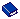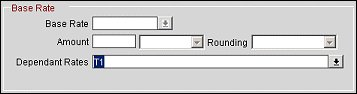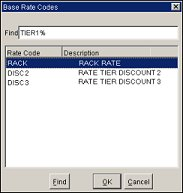Base Rate

(Available in PMS and ORS.)

There are two base rate application settings: Base Rates and Base Rate Extra Person Calculation.

Note: Dynamic Base Rates is additional base rate functionality outside of the "traditional" Base Rate functionality. Advanced Dynamic Base Rates, also discussed below, is an even more complex, yet comprehensive, rate tool also outside of the "traditional" Base Rate functionality.

#### Base Rates

When the Rates>Base Rates application function is set to Y, a rate code may be derived from another (master) rate code, called the base rate. The rate code that derives its rates from the base rate is called the "dependent rate" and will appear in the Dependent Rates field on the More tab (visible when a Base Rate is selected having dependent codes).

Note: Up to 75 dependent rate codes can be based off of a given base rate code. For example, if rate code RACK is a base rate code, rate codes CORP1 through CORP75 can be based off of RACK. However, if you attempt to add CORP76 as a dependent rate code based off of RACK, you will receive the message: "A maximum of 75 rates can be attached to a base rate." If the hotel already has rate codes with more than 75 dependent rates prior to installing/upgrading OPERA, the existing count will be honored but new dependent rates cannot be added to these master base rate codes.

When you select a base rate code from the Base Rate LOV, its rate details will auto-populate into the current rate code's rate details (making the current rate code a dependent rate code). The current rate code is identified in the Rate Code field in the Search criteria. Any changes in the base rate's rate details will automatically affect dependent rate codes for which it is the base rate. The Amount and Rounding fields allow you to vary the rates for the current rate code by a percentage or flat amount over or under the base rates. When a rate code is based on another rate, its rate details are restricted; the base rate controls them automatically. This feature is useful, for example, where you have negotiated rates that are a certain percentage off of the property's RACK rate code. In the event that you have multiple negotiated rates all with different percentages of RACK, it is easy to manage rate changes. When RACK rates change, the rates based on it will automatically change.

When using Base Rates, rates will only be populated on the dependent rate for those room types defined on the (dependent and base) Rate Header.

For example, RACK rate code has the below room types configured:
SEAQN
BHUQB
POKB
POQB

DEP rate code has the below room types configured:
SEAQN
7KN

When performing a Rate Query for DEP rate code, rate amounts will be shown for SEAQN room type only. All other room types will be null.

The Base Rates function activates the Base Rate section on the More tab of the Rate Setup screen.Provide the following information and select the Save button.

Base Rate. Select the down arrow to display a Base Rate Codes list of values and choose the rate code on which the rate details for the current rate code are to be based. (The current rate code is identified in the Rate Code search field.)

Note: The Base Rate list of values field becomes inactive when one or more Dependent Rates have been defined.

Note: If the current rate code (the rate code shown in the Rate Code field at the top of the screen) is marked as a Daily Rate rate code, the Base Rate field LOV will display only other rate codes marked as daily rates.

Note: If the current rate code (the rate code shown in the Rate Code field at the top of the screen) is the master rate code for a dependent rate code, the Base Rate field is not available. For example, assume rate code B is based off of rate code A (A is the master, B is dependent). The Base Rate field on the More tab for rate code A will be unavailable.

The Base Rate LOV includes rate codes that are marked as Best Available Rate (BAR) codes on their rate headers and tiered rate codes if the rate code being configured is itself tiered. Rate codes marked on their rate headers as Daily Rates are excluded. (See Technical Note: Daily Rates and Base Rates, below.) Also excluded are rate codes based off of other rate codes. For example, assume rate code B is based off of rate code A. When creating new rate code C and selecting the Base Rate LOV, rate code A will be available but rate code B will not.

This also means that the Base Rate LOV will exclude any rate code that is configured with a Dynamic Base Rate rate code or an Advanced Dynamic Base Rate rate code.

Note: When a rate is defined as tiered (available when application function Rates>Rate Tiers is set to Y) on its rate header, the Base Rate LOV on the More tab will display only those rates which are also tiered. When the rate is not defined as tiered, the LOV for the Base Rate will only display those rates which are not tiered.

Note: If the dependent rate is un-attached from the base rate, the dependent rate retains its rate details, which then become editable.

Technical Note - Daily Rates and Base Rates: A dependent rate code cannot be based off of a Daily Rate rate code due to storage and performance reasons. Because the dependent rate code stores a complete set of rate details that is based off of the Base Rate's rate details, the rate details of a rate code based off a Daily Rate rate code would need to match a potentially large number of rate details because the Daily Rate rate code stores rate details for every individual day. Moreover, every time the Base Rate's rate details change, the rate details for the dependent rate must also change. An update to a Daily Rate rate code, which stores rates for each individual day, can affect, perhaps, hundreds of Daily Rate rate details. If the Daily Rate rate code were allowed as a Base Rate, the rate details for all dependent rates based on that Daily Rate would also be automatically updated, triggering a potentially great amount of database activity. Dynamic Base rates and Advanced Dynamic Base Rates, on the other hand, do not physically store rate details keyed off of the rate code selected as their Base Rate. These types of rate codes can, therefore, be dependent rate codes based off of Daily Rate rate codes without impacting storage or performance.

Amount. In the first field, enter the flat amount or percentage value. Select the down arrow to choose Flat Amount or Percentage, depending on how you wish to calculate the rate difference between the base rate and the current rate. A decimal amount can also be entered.

Note: The Amount field becomes inactive when one or more Dependent Rates have been defined.

Rounding. Select the down arrow and choose Up, Down, None, Up-Keep Decimal, or Down-Keep Decimal depending on how you wish to round the calculated rates.

• Up. Rounds up to the next highest whole number. (12% x 110 = 13.20. Rounded up = 14)
• Down. Rounds down to the next lowest whole number. (12% x 110 = 13.20. Rounded Down = 13)
• None. Does not round.
• Up-Keep Decimal. Keeps the decimal increments, while rounding up to the next highest whole unit.
Example:
Base rate = \$115.95
Child rate = 10% off Base rate
115.00 / 10% = 103.50
Rounded Up-Keep Decimal = 104.00
Child Rate (BAR) = \$104.95

Note: The child rate, which is the BAR rate, retains the .95 rate plan. This allows you to offer the lowest rate (even if by .05) and work the .95 psychological pricing structure.

• Down-Keep Decimal. Keeps the decimal increments, while rounding down to the next lowest whole unit.
Example:
Base rate = \$115.95
Child rate = 10% off Base rate
115.00 / 10% = 103.50
Rounded Down-Keep Decimal = 103.00
Child Rate (BAR) = \$103.95

Note: The child rate, which is the BAR rate, retains the .95 rate plan. This allows you to offer the lowest rate (even if by .05) and work the .95 psychological pricing structure.

Note: The Rounding field becomes inactive when one or more Dependent Rates have been defined.

Dependent Rates. This field is visible when rates have been configured as being dependent on the base rate code. This drop down list is for information purposes only. The base rate code is displayed in the Rate Code field in the Search criteria. Should a dependent rate need to be removed, then the More tab for the dependent rate can be selected and the base rate code can be removed from the Base Rate Code field, along with the base rate calculation attributes.

When viewing a dependent rate code that is configured to be based off of another base rate code, the Rate Detail tab will be similar to its base rate with the calculations taken into consideration. The Rate Details tab for the dependent rate code cannot be directly modified.

Note: When a package is attached to a BAR rate, it will only be included in rate amount while querying availability for the actual BAR rate and not the dependent rate.

Note: When one or more Dependent Rates are defined, the Base Rate, Amount, and Rounding fields become inactive.

#### Base Rate Extra Person Calculation

When the Rates>Base Rate Extra Person Calculation application parameter is set to Y, base rate calculations will be applied to extra adult and child rate amounts. When the parameter is set to N, the extra adult or child amount will not be discounted by the amount set on the dependent rate. All amounts are calculated based on the status of the application parameter at the time the rate record is created/updated. Changes made to the application parameter (Y/N) will affect all rate records created from that point forward (previously created rate records will not be dynamically updated simply by setting the parameter to Y or N). When an existing record is modified, the status of the application will be verified with the rate amounts being calculated accordingly.

Example 1: Base Rate Extra Person Calculation set to N

Rate Code A (Base Rate) amounts are as follows:

Rate Code B (Dependent Rate) rate amounts are 25% off of the Base Rate. Rate amounts would be as follows for 1 night:

*Extra Adult amount not discounted when Base Rate Extra Person Calculation is set to N.

Example 2: Base Rate Extra Person Calculation set to Y

Rate Code A (Base Rate) rate amounts are as above. Rate Code B (Dependent Rate) rate amounts are 25% off of the Base Rate. Rate amounts would be as follows for 1 night:

 No. Persons Dependent Rate Amount Dependent Rate Calculation 1 Adult 75 100 -25 2 Adults 150 200 -50 3 Adults 187.50 250 -62.50* 4 Adults 225 300 -75* 5 Adults 262.50 350 -87.50*

*When Base Rate Extra Person Calculation is set to Y, rate amount = calculated Rate Code A (Base Rate) amount minus the 25% Dependent Rate discount.

#### Base Rate Codes, Dependent Rate Codes and Packages

Dependent rate codes can have packages added to their rate header, but not to their rate details. For example, if Rate Code B is a dependent rate code based on Rate Code A, packages may be manually added to Rate Code B's header, but not to its rate details. Rate Code B can have a package attached to its rate details only if the package has been copied from Rate Code A's rate details. Moreover, if Rate Code B has a package associated with its rate details, the package cannot be removed or modified because it is derived from Rate Code A's rate details.

Furthermore, if Rate Code A has a package with an allowance attached to its rate details, and Rate Code B—which is dependent on Rate Code A — is attached to an in-house reservation that has the allowance for this package, the package may not be modified or removed from Rate Code A.

#### Using the Repeat Button with Dependent Rate Codes

The button may be used to duplicate a rate code header and, optionally, the rate details of a selected rate code in order to create one or more new rate codes to be configured for the current property. (Use of this function button is described in the Rate Header Tab topic.) When is used with a dependent rate code, and the Copy Rate Details check box is selected, the rate details will be copied to the new rate code but, unlike for the dependent rate code itself, the rate details will be editable. In addition, because the new rate code is not dependent on another rate code, the Base Rate, Amount, and Rounding fields on its More tab will be blank.

#### Tiered Rates and Base Rates

When the Base Rate rate code is itself a tiered rate code (that is, when the Tiered check box is selected on the Rate Header tab), the Base Rate LOV displays only tiered rate codes for selection as the Base Rate. When the Base Rate rate code is selected for a rate query, the rate display will apply the rate calculation to the base rate for the appropriate length of stay. For example, TIER1 is a Tiered Rate rate code with the following LOS tiers:

 LOS Tier Rate Tier 1 (1 night) 200 Tier 2 (2-3 nights) 150 Tier 3 (4-5 nights) 100 Tier 4 (6 or more nights) 50

If ETIER2 is a Base Rate rate code, also a Tiered Rate rate code, that is based off of ETIER1, and ETIER2's rate calculation is -25 flat off of the ETIER1 rate, the following rates would apply depending on the length of stay:

 LOS Tier Rate Tier 1 (1 night) 175 Tier 2 (2-3 nights) 125 Tier 3 (4-5 nights) 75 Tier 4 (6 or more nights) 25

When the Tiered check box is selected on the Rate Header tab and the RATE>RATE TIERS CALCULATION application setting is set, the Base Rate LOV displays rate codes that also have the Tiered check box selected:Example 1: When the application setting is set to DAILY, OPERA will perform the calculation gradually based on each night within the Tier.

RACK rate code is configured with the following Rate Tier amounts: 08/23 = \$100.00, 08/24 = \$100.00, 08/25 = \$100.00, 08/26 = \$95.00, 08/27 = \$95.00. DISC1 rate code is based off RACK: -\$5.00.

The guest requests rates for a 5 night stay, DISC1 rate code.

The rates displayed are as follows: 08/23 = \$95.00, 08/24 = \$95.00, 08/25 = \$95.00, 08/26 = \$90.00, 08/27 = \$90.00

Example 2: When the application setting is set to ENTIRE STAY, OPERA will use the set fixed rate for the entire stay.

Using the same Rates as above, the guest requests rates for a 5 night stay, DISC1 rate code.

The rates displayed are as follows: 08/23 = \$90.00, 08/24 = \$90.00, 08/25 = \$90.00, 08/26 = \$90.00, 08/27 = \$90.00

OPERA will look at the nights for the entire stay and display the rate amount in the last tier, in this example, \$90.00.

Example 3: When the application setting is set to FIRST TIER, OPERA will use the rate amount of the first tier, regardless of the length of stay.

Using the same Rates as above, the guest requests rates for a 5 night stay, DISC1 rate code.

The rates displayed are as follows: 08/23 = \$95.00, 08/24 = \$95.00, 08/25 = \$95.00, 08/26 = \$95.00, 08/27 = \$95.00.

Opera will look at the rate amount for the first tier only, in this example, \$95.00.